# Mensuration

Mensuration is the branch of mathematics that studies the measurement of geometric figures and their parameters like length, volume, shape, surface area, lateral surface area, etc. Learn about mensuration in basic Mathematics.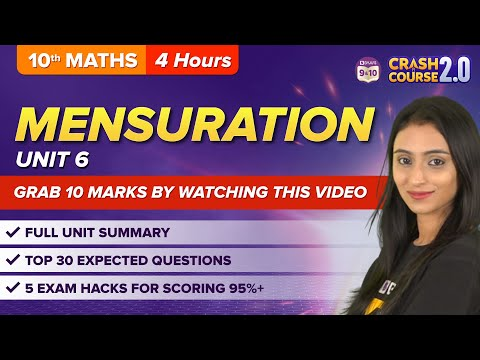Here, the concepts of mensuration are explained and all the important mensuration formulas are provided. Also, the properties of different geometric shapes and the corresponding figures are given for a better understanding of these concepts.

## Mensuration Maths- Definition

A branch of mathematics that talks about the length, volume, or area of different geometric shapes is called Mensuration. These shapes exist either in 2-dimensions or 3-dimensions. Let’s learn the difference between the two.

Differences Between 2D and 3D shapes

2D Shape 3D Shape
If a shape is surrounded by three or more straight lines in a plane, then it is a 2D shape. If a shape is surrounded by a no. of surfaces or planes then it is a 3D shape.
These shapes have no depth or height. These are also called solid shapes and unlike 2D they have height or depth.
These shapes have only two dimensions say length and breadth. These are called Three dimensional as they have depth (or height), breadth and length.
We can measure their area and Perimeter. We can measure their volume, Curved Surface Area (CSA), Lateral Surface Area (LSA), or Total Surface Area (TSA).

## Mensuration in Maths- Important Terminologies

Let’s learn a few more definitions related to this topic.

 Terms Abbreviation Unit Definition Area A m2 or cm2 The area is the surface which is covered by the closed shape. Perimeter P cm or m The measure of the continuous line along the boundary of the given figure is called a Perimeter. Volume V cm3 or m3 The space occupied by a 3D shape is called a Volume. Curved Surface Area CSA m2 or cm2 If there’s a curved surface, then the total area is called a Curved Surface area. Example: Sphere Lateral Surface area LSA m2 or cm2 The total area of all the lateral surfaces that surrounds the given figure is called the Lateral Surface area. Total Surface Area TSA m2 or cm2 The sum of all the curved and lateral surface areas is called the Total Surface area. Square Unit – m2 or cm2 The area covered by a square of side one unit is called a Square unit. Cube Unit – m3 or cm3 The volume occupied by a cube of one side one unit

## Mensuration Formulas

Now let’s learn all the important mensuration formulas involving 2D and 3D shapes. Using this mensuration formula list, it will be easy to solve the mensuration problems. Students can also download the mensuration formulas list PDF from the link given above. In general, the most common formulas in mensuration involve surface area and volumes of 2D and 3D figures.

## Mensuration Formulas For 2D Shapes

Below are the mensuration formulas for two-dimensional shapes in geometry.

Shape Area (Square units) Perimeter (units) Figure
Square a2 4a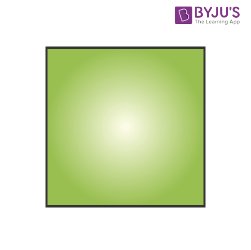Rectangle l × b 2 ( l + b)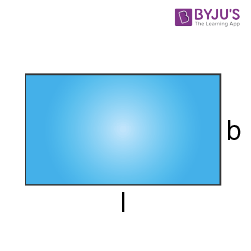Circle πr2 2 π r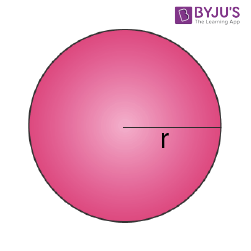Scalene Triangle √[s(s−a)(s−b)(s−c)],

Where, s = (a+b+c)/2

a+b+c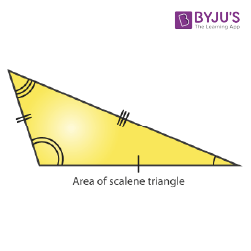Isosceles Triangle ½ × b × h 2a + b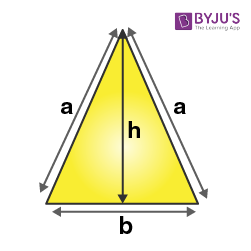Equilateral triangle (√3/4) × a2 3a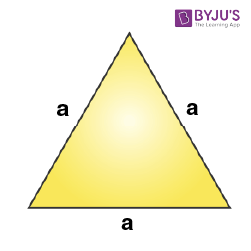Right Angle Triangle ½ × b × h b + hypotenuse + h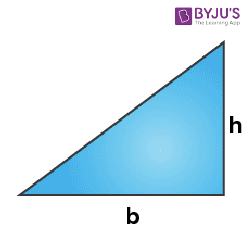Rhombus ½ × d1 × d2 4 × side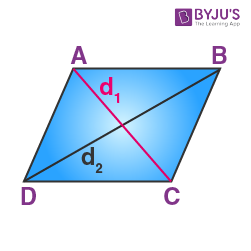Parallelogram b × h 2(l+b)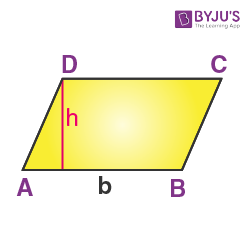Trapezium ½ h(a+c) a+b+c+d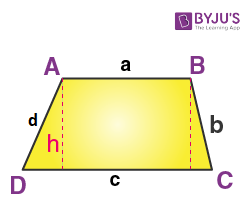## Mensuration Formulas for 3D Shapes

Go through the mensuration formulas tabulated below for three-dimensional shapes in geometry.

Shape Volume (Cubic units) Curved Surface Area (CSA) or Lateral Surface Area (LSA) (Square units) Total Surface Area (TSA) (Square units) Figure
Cube a3 LSA = 4 a2 6 a2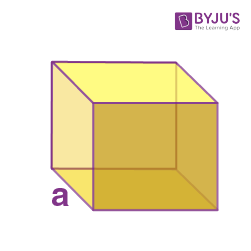Cuboid l × b × h LSA = 2h(l + b) 2 (lb +bh +hl)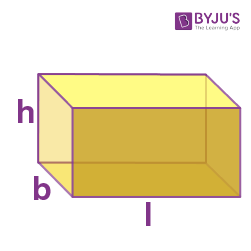Sphere (4/3) π r3 4 π r2 4 π r2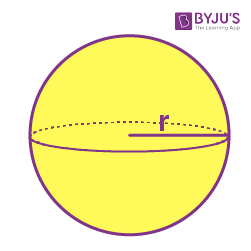Hemisphere (⅔) π r3 2 π r 2 3 π r 2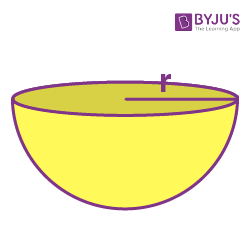Cylinder π r 2 h 2π r h 2πrh + 2πr2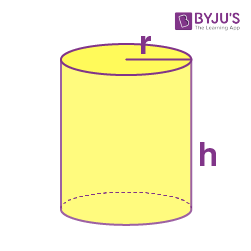Cone (⅓) π r2 h π r l πr (r + l)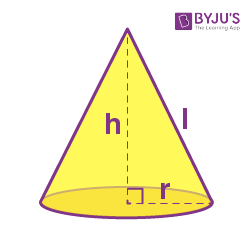## Mensuration Solved Problems

### Question 1:

Find the area and perimeter of a square whose side is 5 cm.

Solution:

Given:

Side = a = 5 cm

Area of a square = a2 square units

Substitute the value of “a” in the formula, we get

Area of a square = 52
A = 5 × 5 = 25

Therefore, the area of a square = 25 cm2

The perimeter of a square = 4a units

P = 4 × 5 =20

Therefore, the perimeter of a square = 20 cm.

Question 2:

What is the circumference of a circle with a radius of 3.5 cm?

Solution:

Given,

Radius of the circle = r = 3.5 cm

We know that,

Circumference of a circle with radius r = 2πr

Substituting r = 3.5 cm in the above formula, we get;

= 2 × (22/7) × 3.5

= 22 cm

Hence, the circumference of the circle is 22 cm.

Question 3. Find the area of an equilateral triangle whose altitude is given by (√3/2) cm.

Solution: Given,

The altitude of equilateral triangle = √3/2 cm

The formula for altitude of equilateral triangle is given by √3/2 (side)

Hence, on comparing, we get;

√3/2 = √3/2 (side)

side = 1 unit

So,

Area of equilateral triangle = √3/4 (side)2

= √3/4 (1)2

= √3/4 sq.cm.

Required, area of the triangle is √3/4 square cm.

### Practice Questions

1. Calculate the area of the rectangle whose length is 22 cm and breadth is 17 cm.
2. Find the volume of a right circular cylinder with a base radius of 14 cm and a height of 10 cm.
3. What is the surface area of a cube of edge 18 m?

## Frequently Asked Questions on Mensuration

Q1

### What is mensuration in Maths?

In maths, mensuration is defined as the study of the measurement of various 2D and 3D geometric shapes involving their surface areas, volumes, etc.

Q2

### What is the difference between mensuration and geometry?

Mensuration refers to the calculation of various parameters of shapes like the perimeter, area, volume, etc. whereas, geometry deals with the study of properties and relations of points and lines of various shapes.

Q3

### What are 2D and 3D Mensuration?

2D mensuration deals with the calculation of various parameters like the area and perimeter of 2-dimensional shapes like squares, rectangles, circles, triangles, etc.

3D mensuration is concerned with the study and calculation of surface area, lateral surface area, and volume of 3-dimensional figures like a cube, sphere, cuboid, cone, cylinder, etc.

1. TRULY INFORMATIVE AND HELPFUL. THANK YOU BYJU’S.

2. Very good but an you give NCERT surface area volume 9th standard questions

3. Byju’s is an awesome app. It helps me a lot. Thank you Byju’s

4. It was very helpful for me

5. very informative site for Maths.

6. its really healpful

7. Thanks guys, you saved me from my exam tomorrow

8. I want to learn mensuration

9. Byju’s is a great app Thank you so much

10. Mujhe sir notes chalice.

11. very good sir but there is no pyramid formulae

12. very efficient!!
keep it up

13. Byju’s is an awesome app. It helps me a lot. Thank you Byju’s. Byju’s is a great app very informative site for all subject. Very helpful. Thank you so much. I like this app.